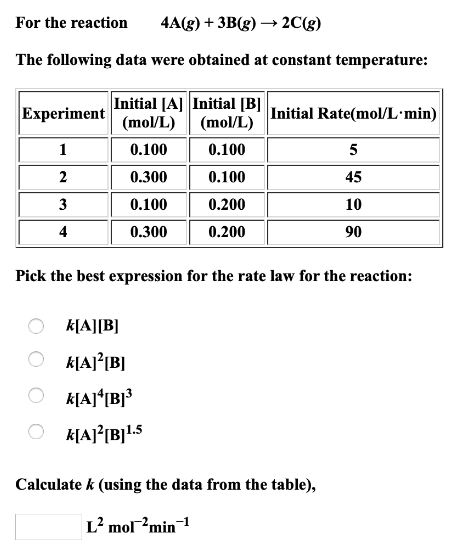# For the reaction 4A(g) + 3B(g) → 2C(g) The following data were obtained at constant temperature: Pick the best expression for the rate law for the reaction: a) k[A][B] b) k[A]^2[B] c) k[A]^4[B]^3 d) k[A]^2[B]^1.5 Calculate k (using the date from the table),